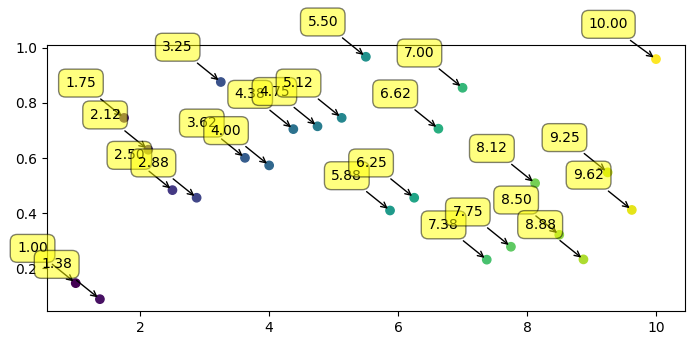# How to annotate the points on a scatter plot with automatically placed arrows in Matplotlib?

To annotate the point on a scatter plot with automatically placed arrows, we can take the following steps −

• Create points for x and y using numpy.

• Create labels using xpoints.

• Use scatter() method to scatter the points.

• Iterate labels, xpoints and ypoints and annotate plot with label, x and y with different properties.

• To display the figure, use show() method.

## Example

import numpy as np
from matplotlib import pyplot as plt
plt.rcParams["figure.figsize"] = [7.00, 3.50]
plt.rcParams["figure.autolayout"] = True
xpoints = np.linspace(1, 10, 25)
ypoints = np.random.rand(25)
labels = ["%.2f" % i for i in xpoints]
plt.scatter(xpoints, ypoints, c=xpoints)
for label, x, y in zip(labels, xpoints, ypoints):
plt.annotate(
label,
xy=(x, y), xytext=(-20, 20),
textcoords='offset points', ha='right', va='bottom',
plt.show()